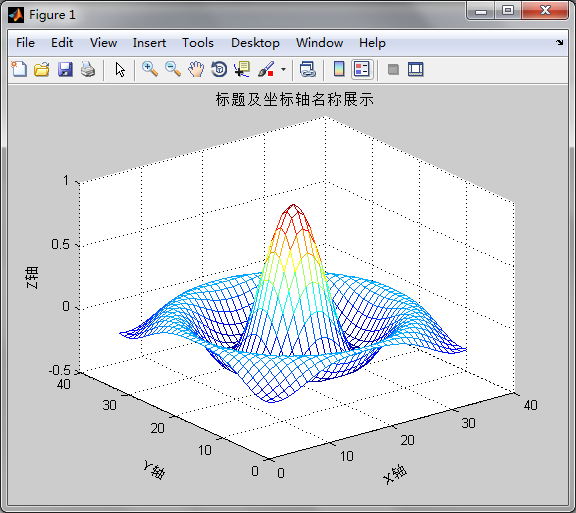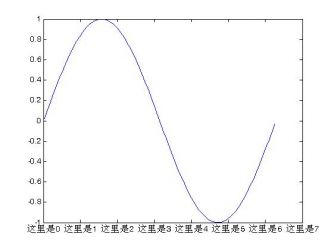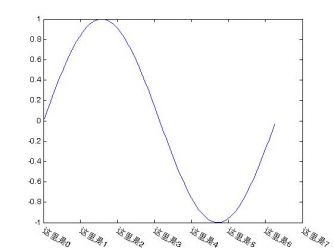• Matlab坐标轴标注和特殊字体

万次阅读 2018-05-02 14:35:05
坐标轴标注：1. 控制坐标轴尺度长度：set(gca,'XLim',[-pi/2 pi])2. 定制自己想标注的刻度： （1）set(gca,'XTick',[-pi/2:pi/4:pi]) （2）set(gca,'XTickLabel',{'-pi/2' '-pi/4:' '0' 'pi/4' 'pi/2' 'pi*3/4' ...

坐标轴标注：

1. 控制坐标轴尺度长度：set(gca,'XLim',[-pi/2 pi])

2. 定制自己想标注的刻度：

1set(gca,'XTick',[-pi/2:pi/4:pi])

2set(gca,'XTickLabel',{'-pi/2' '-pi/4:' '0' 'pi/4' 'pi/2' 'pi*3/4' 'pi'})

3. 反转坐标轴：set(gca,'XDir','reverse')

4. 上下标及latex公式

输下标：_{wb}

输上标：^{0.5}

latex公式：
text('Interpreter','latex',...
'String','$$\int_0^x\!\int_y dF(u,v)$$',...
'Position',[.5 .5],...
'FontSize',16)

Matlab中输入上标、下标、特殊符号或字体：

bf\it\rm表示黑体，斜体，正体字符

上标用 ^(指数)，下标用 _(下划线)

调用方式为: ^{任意字符}, _{任意字符}, 注意{}的使用!

希腊字母等特殊字符用 \加拼音如:
α \alpha     β \beta         γ \gamma     θ \theta     Θ \Theta
Г \Gamma     δ \delta       Δ \Delta     ξ \xi         Ξ \Xi         Ω \Ommiga
η \elta     ε \epsilong     ζ \zeta       μ \miu       υ \nu         τ \tau
λ \lamda     Λ \Lamda       π \pi         Π \Pi       σ \sigma       Σ \Sigma
φ \phi       Φ \Phi         ψ \psi       Ψ \Psi       χ \chi         ω \ommiga

< \leq       > \geq        不等于 \neq       << \ll     >> \gg

正负 \pm     左箭头\leftarrow     右箭头 \rightarrow     上箭头 \uparrow

体会以下两例:
figure, title('\zeta^{-t}sint');
figure, title('x~{\chi}_{\alpha}^{2}(3)');

matlab设置线条颜色宽度线型

2010-11-02 14:03

 多组变量绘图:plot(x1, y1, 选项1, x2, y2, 选项2, ……)上面的plot格式中,选项是指为了区分多条画出曲线的 颜色,线型及标记点而设定的曲线的属性.MATLAB在多组变量绘图时,可将曲线以不同的颜色,不同的线型及标记点表示出来.颜色属性'r' 红色 'm' 粉红'g' 绿色 'c' 青色'b' 兰色 'w' 白色'y' 黄色 'k' 黑色线型属性'-' 实线 '--' 虚线':' 点线 '-.' 点划线标记点属性'.' 用点号绘制各数据点 '^' 用上三角绘制各数据点'+' 用'+'号绘制各数据点 'v' 用下三角绘制各数据点'*' 用'*'号绘制各数据点 '>' 用右三角绘制各数据点' .' 用'.'号绘制各数据点 '<' 用左三角绘制各数据点's'或squar 用正方形绘制各数据点'p' 用五角星绘制各数据点'd'或diamond用菱 形绘制各数据点 'h' 用六角星绘制各数据点这些选项可以连在一起用,如:'-.g'表示绘制绿色的点划线,'g+'表示用绿色的 '+'号绘制曲线.注意：1)表示属性的符号必须放在同一个字符串中;2)可同时指定2~3个属性;3) 与先后顺序无关;4)指定的属性中,同一种属性不能有两个以上.例:t=0:0.1:2*pi;y1=sin(t);y2=cos(t);y3=sin(t).*cos(t);plot(t,y1, '-r',t,y2, ':g',t,y3, '*b')该程序还可以写成:t=0:0.1:2*pi;y1=sin(t);y2=cos(t);y3=sin(t).*cos(t);plot(t,y1, '-r')hold onplot(t,y2, ':g')plot(t,y3, '*b')hold off注:在MATLAB中,如画图前已有打开的图形窗口,则再画图系统将自动擦掉坐标系中已有的图形对象,但设置了hold on后,可以保持坐标系中已绘出的图形.设置线宽度(LineWidth)，标记点的边缘颜色(MarkerEdgeColor)，填充颜色 (MarkerFaceColor)及标记点的大小(MarkerSize)例:设置绘图线的线型,颜色,宽度,标记 点的颜色及大小：t=0:pi/20:pi;y=sin(4*t).*sin(t)/2;plot(t,y,'-bs','LineWidth',2,... %设置线的宽度为2'MarkerEdgeColor','k',... %设置标记点边缘颜色为黑色'MarkerFaceColor','y',... %设置标记点填充颜色为黄色'MarkerSize',10) %设置标记点的尺寸为10
展开全文• 原文地址：matlab坐标轴标注和特殊字体（转载）作者：残翅天使原文链接地址：http://blog.sina.com.cn/s/blog_4cd90db30100d91s.html 如果这里的不够详尽，另外提供一个链接也很不错 ...坐标轴标注： ...

原文链接地址：http://blog.sina.com.cn/s/blog_4cd90db30100d91s.html

如果这里的不够详尽，另外提供一个链接也很不错

http://wenku.baidu.com/view/092e7a00bed5b9f3f90f1c31.html

坐标轴标注：

1. 控制坐标轴尺度长度：set(gca,'XLim',[-pi/2 pi])

2. 定制自己想标注的刻度：

（1）set(gca,'XTick',[-pi/2:pi/4:pi])
（2）set(gca,'XTickLabel',{'-pi/2' '-pi/4:' '0' 'pi/4' 'pi/2' 'pi*3/4' 'pi'})

3. 反转坐标轴：set(gca,'XDir','reverse')

4. 上下标及latex公式

输下标：_{wb}

输上标：^{0.5}

输latex公式：
text('Interpreter','latex',...
'String','$$int_0^x!int_y dF(u,v)$$',...
'Position',[.5 .5],...
'FontSize',16)

在Matlab中输入上标、下标、特殊符号或字体：

bf，it，rm表示黑体，斜体，正体字符

上标用 ^(指数)，下标用 _(下划线)

调用方式为: ^{任意字符}, _{任意字符}, 注意{}的使用!

希腊字母等特殊字符用 加拼音如:
α alpha     β beta         γ gamma     θ theta     Θ Theta
Г Gamma     δ delta       Δ Delta     ξ xi         Ξ Xi         Ω Ommiga
η elta     ε epsilong     ζ zeta       μ miu       υ nu         τ tau
λ lamda     Λ Lamda       π pi         Π Pi       σ sigma       Σ Sigma
φ phi       Φ Phi         ψ psi       Ψ Psi       χ chi         ω ommiga

< leq       > geq        不等于 neq       << ll     >> gg

正负 pm     左箭头 leftarrow     右箭头 rightarrow     上箭头 uparrow

体会以下两例:
figure, title('zeta^{-t}sint');
figure, title('x~{chi}_{alpha}^{2}(3)');

展开全文• matlab绘图如何添加图例/坐标轴刻度像这个图里的 那样的图例如何添加 还有坐标轴的刻度如何用像上图的序号 首先打开电脑上的“matlab”软件，主界面如下图所示，箭头处输入代码即可运行。下面输入代码绘制图像，...

matlab绘图如何添加图例/坐标轴刻度

像这个图里的 那样的图例如何添加 还有坐标轴的刻度如何用像上图的序号 首先打开电脑上的“matlab”软件，主界面如下图所示，箭头处输入代码即可运行。

下面输入代码绘制图像，命令行代码如下图所示。点击enter键之后，即可运行程序绘制图像，正弦函数图像，可以看到x和y的坐标轴刻度。

MATLAB如何将坐标标注在上部和右侧？

matlab中坐标轴默认在左侧和下部显示，为了将坐标轴移位，可以使用set函数对坐标轴位置进行设定 具体的语句为 set(gca,'xaxislocation','top'); set(gca,'yaxislocation','right'); 其中gca表示当前图像。xaxislocation与yaxislocation表示x与y

MATLAb怎样设置坐标轴

matlab坐标轴如何带单位标注?

X轴单位是度,Y轴的单位是%,如何让坐标轴的数值显示为1度,2度,3度度a=linspace(1,2,10) plot(a,'pr','linewidth',1.5,'MarkerEdgeColor','r','MarkerFaceColor','m','MarkerSize',10) legend('a','Location','best') title('a','FontName','Times New Roman','FontWeight','Bold','FontSize',16) xlabel('T','F

如图所示，运行出来的图形没有坐标轴。

然后点击红框勾选的insert按钮。

如图所示，点击X label按钮。

接着在红框勾选的位置，可以输入X轴的名称。

如图所示，坐标轴设置完成。 注意事项：用MATLAB来解算问题

matlab Y坐标轴的字体如何调大

set(gca, ’XTick’, [0 1 2]) X坐标轴刻度数据点位置 set(gca,'XTickLabel',{'a','b','c'}) X坐标轴刻度处显示的字符 set(gca,'FontName','Times New Roman','FontSize',14)设置坐标轴刻度字体名称，大小 ‘FontWeight’,’bold’ 加粗 ‘FontAngle’,’

Matlab如何在坐标轴上标注希腊字母

举个例子 set(gca,'xtick',0:.5:1,'xticklabel',{'\lambda','\alpha','\sigma'})

matlab 坐标轴上公式如何输入

如图所示，可在图中坐标处直接输入 \it\epsilon^{P}_{\mu}_{max}，但eps我一般用命令来加这个，用的是Latex语句(这个我默认你是能看懂的，不知道怎么弄这个话，你再追问。) 下面是代码： clc;clear;clf;xlabel({'$$\bar \varepsilon _{\mu mean}^P$$'},'Interpreter','latex','FontSize',18)ylabel({'\bar \varep

Matlab坐标轴间隔怎么设定

首先需要打开MATLAB，file-new-script，进行程序编制。

例如这里画一条曲线。”clear;clc;m=1365;a=-11825/m+5.01;x=0:0.1:1.6;b=a-log10(x);y=10.^(b);p=plot(x,y,'r');。

运行程序，可以先保存在运行，进行“save and run”。

展开全文• 利用matlab绘制图形的时候，会遇到坐标轴刻度标签过于拥挤的情况，此时需要将坐标轴刻度标签旋转一个角度，从而在一定程度上消除拥挤状况。本帖中的程序(自编函数rotateticklabel)就是用来实现这个功能(旋转坐标轴...

利用matlab绘制图形的时候，会遇到坐标轴刻度标签过于拥挤的情况，此时需要将坐标轴刻度标签旋转一个角度，从而在一定程度上消除拥挤状况。本帖中的程序(自编函数rotateticklabel)就是用来实现这个功能(旋转坐标轴刻度标签)的。下面给出例子和源代码。

【例】绘制一个周期上的正弦函数图形，将X轴刻度标签修改并旋转。

修改X轴刻度标签的matlab代码：

x = 0:0.05:2*pi;

y = sin(x);

plot(x,y);

str = '这里是0|这里是1|这里是2|这里是3|这里是4|这里是5|这里是6|这里是7';

set(gca,'xtick',0:7,'xticklabel',str);

绘制图形如下，显然X轴刻度标签是比较拥挤的：调用自编函数旋转X轴刻度标签的matlab代码：

rotateticklabel(gca,'x',-30);

旋转后图形如下，拥挤状况得到解决：自编的rotateticklabel 函数代码如下：

function TextHandle = rotateticklabel(ha,tag,rot)

%   旋转坐标轴刻度标签的函数

%   ha   坐标系句柄(默认为当前坐标系)

%   tag  坐标轴标识字符串('X'或'Y')，默认旋转X轴标签

%   rot  旋转角度(单位：度)

%

%   Example:

%   x = 0:0.05:2*pi;

%   y = sin(x);

%   plot(x,y);

%   rotateticklabel(gca,'x',-30);

%

if ~ishandle(ha)

warning('第一个输入参数应为坐标系句柄');

return;

end

if ~strcmpi(get(ha,'type'),'axes')

warning('第一个输入参数应为坐标系句柄');

return;

end

if nargin == 1

tag = 'X';

rot = 0;

elseif nargin == 2

if isnumeric(tag) && isscalar(tag)

rot = tag;

tag = 'X';

elseif ischar(tag) && (strncmpi(tag,'x',1) || strncmpi(tag,'y',1))

rot = 0;

else

warning('输入参数类型错误');

return;

end

else

if ~isnumeric(rot) || ~isscalar(rot)

warning('输入参数类型错误');

end

if ~ischar(tag) || (~strncmpi(tag,'x',1) && ~strncmpi(tag,'y',1))

warning('输入参数类型错误');

end

end

axes(ha);

oldxticklabel = findobj(ha,'type','text','tag','oldxticklabel');

oldyticklabel = findobj(ha,'type','text','tag','oldyticklabel');

if strncmpi(tag,'x',1)

if isempty(oldxticklabel)

str = get(ha,'XTickLabel');

x = get(ha,'XTick');

yl = ylim(ha);

set(ha,'XTickLabel',[]);

y = zeros(size(x)) + yl(1) - range(yl)/30;

TextHandle = text(x,y,str,'rotation',rot,...

'Interpreter','none','tag','oldxticklabel');

else

set(oldxticklabel,'rotation',rot);

TextHandle = oldxticklabel;

end

else

if isempty(oldyticklabel)

str = get(ha,'YTickLabel');

y = get(ha,'YTick');

xl = xlim(ha);

set(ha,'YTickLabel',[]);

x = zeros(size(y)) + xl(1) - range(xl)/10;

TextHandle = text(x,y,str,'rotation',rot,...

'Interpreter','none','tag','oldyticklabel');

else

set(oldyticklabel,'rotation',rot);

TextHandle = oldyticklabel;

end

end

rot = mod(rot,360);

if rot>=0 && rot<180

set(TextHandle,'HorizontalAlignment','right');

else

set(TextHandle,'HorizontalAlignment','left');

end

【咨询联系方式】

QQ号：619492407

电话： (010)68472925(曾老师)

展开全文• Matlab设置自定义和旋转坐标轴刻度值及标签的详细步骤作者:大胡子 来源:PC下载网时间:2020-04-29 10:46:53还不会设置自定义和旋转坐标轴刻度值及标签？其实在Matlab中操作起来是非常方便的。这里笔者就为大伙提供...
• Mathworks Matlab是一款非常专业的数学计算软件，该软件提供...不过很多用户在使用Mathworks Matlab制图后，可能某些坐标轴的位置不是我们想要的，这时我们即可通过命令进行修改，下面看详细的修改方法吧！大小：75....
• matlab plot函数 坐标轴标注

千次阅读 2020-02-17 19:45:16
坐标轴标注 https://jingyan.baidu.com/article/da1091fb1770a1027849d692.htmlmatlab plot
• Matlab绘图坐标轴的设置教程

千次阅读 2021-04-18 09:44:48
编程，绘图，设置坐标轴，做出自己的坐标轴1. axis([xmin xmax ymin ymax])设置当前图形的坐标范围，分别为x轴的最小、最大值，y轴的最小最大值2. V=axis返回包含当前坐标范围的一个行向量3. axis auto将坐标轴刻度...
• 坐标轴标注：1. 控制坐标轴尺度长度：set(gca,'XLim',[-pi/2 pi])2. 定制自己想标注的刻度：(1)set(gca,'XTick',[-pi/2:pi/4:pi])(2)set(gca,'XTickLabel',{'-pi/2' '-pi/4:' '0' 'pi/4' 'pi/2' 'pi*3/4' 'pi'})3. ...
• 1、图形标题设置、及标题的字号、字体例如： title([‘u...2、设置坐标轴字体及字号：set(gca,‘fontsize’,10,‘fontname’,‘Times New Roman’);设置坐标轴上下限：axis([xmin,xmax,ymin,ymax]);也可通过以下方式...
• matlab坐标轴刻度间距

千次阅读 2021-04-25 14:35:30
matlab坐标轴操作大全_电脑基础知识_IT/计算机_专业资料。 坐标轴标注: 1. 控制坐标轴尺度长度:set(gca,'XLim',[-pi/2 pi]) 2. 定制自己想标注的刻度: ......axis normal 自动调整纵横轴比例,使当前坐标轴范围内的...
• matlab提供的默认解释器(Interpreter)为tex，在text的help文档里可以看到各种支持的命令，如希腊字符等，在坐标轴标题里输入根号可以如下命令，在xticklabel下输入可以采用以下命令'ticklabelinterpreter','latex'：...
• MATLAB绘图设置坐标轴标注

万次阅读 2019-07-05 20:51:07
绘图之后设置坐标轴标注 如，set(gca,‘XTickLabel’,{‘1’ ‘2’ ‘3’ ‘4’ ‘5’}); %横轴下标注即被更改为1 2 3 4 5； y轴同理，可按自己需要进行输入。 ...matlab
• 这是一个用于轴标签对齐的简单 MATLAB 函数。 当投影模式为 Perspective 或 DataAspectRatio 不是 [1 1 1] 时，它仍然有效。 ＃＃ 职能- align_axislabel：将$x$、$y$和$z$标签旋转到其对应轴的方向，并将标签移动...matlab
• 从图中我们可以看到，在y轴出现了要求中文为8pt宋体，英文为8ptEculid,有些期刊的英文要求为Times New Roman字体，但是在MATLAB中，对坐标轴调整字体，默认是对坐标轴的所有文字都设置为一种字体。如图2所示： ...matlab ieee论文
• [MATLAB]设置坐标轴标签

千次阅读 2020-05-08 11:27:57
[MATLAB]设置坐标轴标签 >> x=0:0.1:2*pi; >> y=sin(x); >> plot(x,y); >> xlabel('x轴示范','color','b','fontsize',18); >> ylabel('y轴示范','color','r','fontsize',18); 运行...matlab
• matlab坐标轴字体加粗

千次阅读 2021-02-07 02:50:22
matlab画图设置(坐标轴、曲线、颜色)_计算机软件及应用_IT/计算机_专业资料。matlab 画图设置(坐标轴、曲线、颜色) a=linspace(1,2,10) plot(a,'--pr','......(gca,'FontName','Times New Roman','FontSize',14)设置...
• matlab坐标轴范围的设置方法是：1、打开matlab，输入“x=0:0.1:100；y=sin(x)+100；plot(x，y)”画出一个正弦函数图像。2、可以看到x轴显示了从0到100的范围，但是这样导致图像很难看，同时y轴显示的不是从0开始的...
• function th=rotateticklabel(h,rot,demo) ... %X刻度标注字体大小 else th=text(b,repmat(c(1)-.1*(c(2)-c(1)),length(b),1),a,'HorizontalAlignment','left','fontsize',10,'fontweight','bold','rotation',rot); end
• MATLAB 没有任何用于将坐标轴围绕原点居中的内置功能。 此工具是解决此问题的第一个修复程序。 包含一个示例脚本，显示如何使用该功能。 在 MATLAB 2007b 上测试。 工作正常，只要在调用函数后不调整图形大小。matlab
• 注：'gca’可以理解为'get the current axis'的缩写；... 控制坐标轴尺度长度：set(gca,'XLim',[-pi/2pi])2. 定制自己想标注的刻度：(1)set(gca,'XTick',[-pi/2:pi/4:pi])(2)set(gca,'XTickLabel',{'-pi/2''-pi/4:...
• 其中设置内容较多，主要包括：刻度标签的显示刻度标签的显示间隔刻度标签旋转的角度刻度标签的内容格式器刻度标签文字的相关设置parallelAxis.axisLabel | Object坐标轴刻度标签的相关设置。parallelAxis.axisLabel....
• Matlab可以利用简单的命令来改变坐标轴标签的角度、字体、大小、内容等，请不要被那些过时的帖子浪费时间了！ 比如下面的这几个帖子： matlab中figure的坐标轴label、title、xticklabel的旋转 MATLAB 画柱状图（/...matlab
• function th=rotateticklabel(h,rot,size,~)%ROTATETICKLABEL rotates tick labels% TH=ROTATETICKLABEL(H,ROT) is the calling form where H is a handle to% the axis that contains the XTickLabels that are...
• 去掉y数值标注 set(gca,'ytick',[]); 去掉x数值标注 set(gca,'xtick',[]);matlab
• 直白说明： xlabel('{\itx}/m') 出现的结果： x/m 也就是说： {\itx}将x斜体化了~ 明白了吧~matlab xlabel
• 自定义沿坐标轴的刻度值和标签有助于突出显示数据的特定方面。以下示例说明一些常见的自定义，例如修改刻度值的放置位置、更改刻度标签的文本和格式，以及旋转刻度标签。 更改刻度值位置和标签 创建 x，将其指定为...matlab 开发语言
• matlab手动指定坐标轴位置和标签 XTick，XTickLabelmatlab
• matlab绘图如何定义坐标轴上的数字字体大小？方法一：在画完图的Figure窗口里，通过菜单Edit-Axes Properties....-Font-Font Size即可设置。=================================================================......

matlab坐标轴标签matlab 订阅